# Types of force

## Two types of force:

• Contact Force,
• Non-Contact Force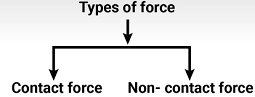• CONTACT FORCE: Force which acts on a body only when the body is in contact with the force is known as contact force. In other words, the forces which act on a body either directly or through some connector are called contact forces.
e.g. Biological forces, mechanical forces and frictional forces are the examples of contact forces.

### Types of contact force:

•  Normal force
•  Force of friction

### Normal force:

If contact forces between the bodies are perpendicular to the surface in contact, the forces are known as normal forces.

e.g.  Consider a book on a table. The table pushes the book upwards and book pushes the table downwards, these forces are perpendicular to the surfaces of book and table. Thus the table applies a normal forces on book in the upward direction and book applies a normal force on table in downward direction.

### (a) Biological force or muscular force

The force which we apply by our muscles to do our work for example, to lift the school bag, to kick a football, to move or to run is known as muscular force. When we lift, push, pull, stretch or compress something, we use our muscular force. For example, lifting of a heavy weight, pulling of a wheel chart, compressing a bale or cotton involves muscular force. Blood gets pushed into our arteries by the muscular force exerted by our heat. We also use the muscular force of the animals. For example, a horse uses muscular force to pull a cart, bullocks are used for ploughing fields.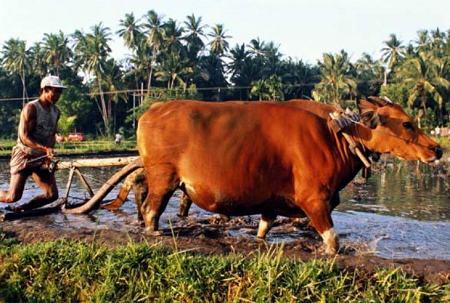Bullocks ploughing field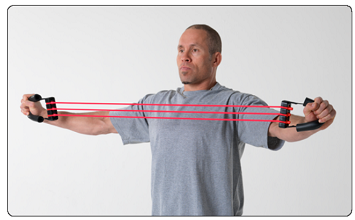A man stretching chest expander

This force produced by the muscles of living beings is called muscular force or biological force.

### (b) Mechanical forces

The forces generated by a machine are called mechanical forces.

The force used to run a motor car engine is produced by using the energy of petrol. The force used to run steam engine is produced by using the energy of coal.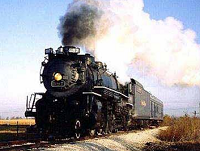Steam engine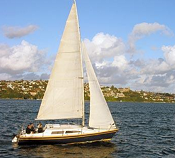A sail boat

### (ii) Force of friction:

Two bodies placed in contact can also exert forces parallel to the surfaces in contact, such a force is called force of friction or simply friction.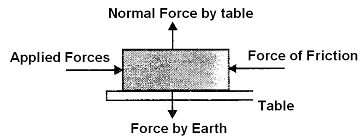Suppose a body is placed on the table following three forces act on it:

• (A) Force by earth in downward direction.
• (B) Normal force due to table in upward direction.
• (C) Applied force towards right.

Body is not moving, so all the forces must be balanced, normal force by table and force by earth balanced each other, to balance the applied force there must be an equal and opposite force, this force is known as force of friction. If we increase the applied force and the body is still at rest it means force of friction also increases till it balanced by the applied force. The force of friction is self adjusting force. On increasing the applied force the force of friction will increase upto a limit. It is known as limiting friction, after it on increasing the applied force, the body starts to move.

 We also know that force can either slow down or stop the moving bodies. This force which stops the moving bodies is called force of friction. It is produced when an object moves in contact with another object.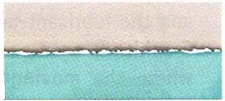A force which acts at the surface of contact, when one body moves or tends to move upon another body is called the force of friction. It is a force that slows things down or prevents things from moving.

### TENSION:

When a body is connected by means of a string or a rope, a force may be exerted on the body by the string or the rope. This force is called tension.

To find the direction of tension, remember this point Tension force always pulls a body”.

eg.

Regarding the tension and string, the following three points are important to remember:

• 1. If a string is inextansible the magnitude of acceleration of any number of masses connected through string is always same.
• 2. If a string is massless, the tension in it is same everywhere, however, if a string has a mass, tension at different points will be different.
• 3. If there is friction between string and pulley, tension is different on two sides of the pulley, but if there is no friction between pulley and string tension will be same on both sides of the pulley.• NON-CONTACT FORCE: Force which acts on a body when the body is not in contact with the force is known as non-contact force.

### Types of non-contact force:

• Gravitational force
• Electrostatic/ Electric force
• Magnetic force
• Gravitational force : Gravity is the force with which the earth pulls everything towards itself. It is due to gravity that things on earth fall downwards and not upwards.
The pull exerted by the earth on the objects is called force of gravity or gravitational force.
Whenever an object is dropped, it always falls towards the ground. For example, a ball thrown up falls down towards the earth ripe fruits on a tree fall towards the ground. A stone on the top of a hill falls downward, if pushed.
It was Newton, who said that every object in this universe attracts every other object with a certain force. The force with which two objects attract each other is called the force of gravitation. The force of gravitation acts even if the two objects are not connected by any means. If, however, the masses of the objects are small, the force of gravitation between them is small and cannot be detected easil
The force of attraction between any two particles in the universe is called gravitation or gravitational force.

### Force of gravity :

The earth attracts all the bodies towards its centre. The force exerted by the earth on the body is known as weight of the body or force of gravity. It acts in vertically downward direction.

If mass of the body is m and acceleration due to gravity is g. Then force of gravity or weight = mg. The value of g is 9.8 m/s2. For a body moving downward, g is taken as positive while for a body moving upward, g is taken as negative.

It is the force of gravity which gives weight to an object. Weight is basically the force of gravity acting on a body.

The force (or pull) by which an object is attracted towards earth is called weight.

 To show that weight of a body is a force. Material required: Strip of rubber,20 cm long, 2 cm wide, a pin, a heavy stone, a table. Method: Fix the rubber strip to the edge of a table with a drawing pin as shown in figure. Now pull the rubber in the downward direction with your hand as shown in figure (b).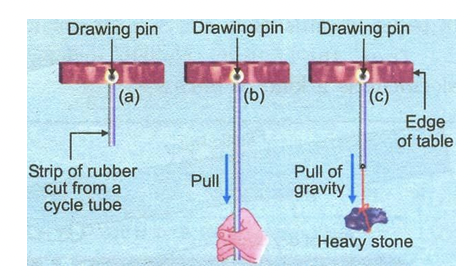You will see that rubber gets stretched. Thus, the force (pull) causes stretching in the rubber tube. Now suspend a heavy stone from the rubber strip with the help of a strong cotton thread as shown in figure (c). It is seen that rubber strip gets stretched. What causes this stretching? Obviously, it is the weight of the stone which causes stretching, similar to the stretching caused by pulling it. This activity clearly proves that weight is a kind of force.

### Spring Balance :

Spring balance is a device used for measuring the force acting on an object. It consists of a coiled spring which gets stretched when a force is applied to it. Stretching of the spring is measured by a pointer moving on a calibrated scale. The reading on the scale gives the magnitude of the force.

• Electrostatic and Electric force : Electrostatic force is another kind of force which can push or pull tiny objects. The following activity will prove it. To show electrostatic force attracts tiny bits of paper.
• Material required: Small bits of dry paper a plastic ruler.
• Method: Rub one end of the plastic ruler against your dry hair or woollen cloth. Bring the rubbed end of the ruler near tiny bits of paper. The tiny paper bits cling to the plastic ruler as shown in the figure.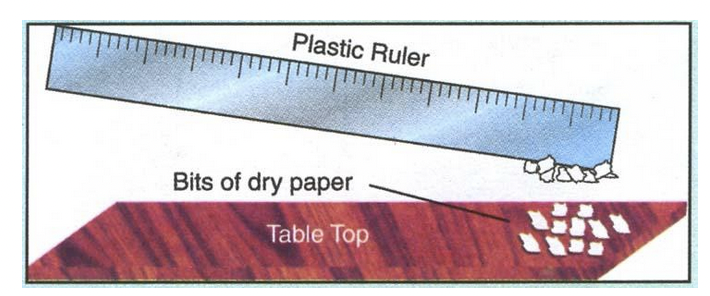• Reason: This happens because the plastic ruler gets electrically charged when rubbed with hair or wool. The electricity so produced pulls the bits of paper towards itself.
• This activity clearly proves that electricity is kind of force.
•  The electrostatic force is used in removing carbon and ash particles in smoke, coming out of the chimneys of big factories. This helps in reducing air pollution

### Electric Force:

The force acting between the charges is known as electric force. If q1 and q2 are the charges then the electric force acting between them would be,

F =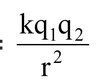, k = 9 x 109 Nm2/C2

where r is the distance between q1, and q2, k is the Coulomb's constant.

Force can be attractive or repulsive.

• Magnetic force : The strange property of some substances to attract iron or steel objects towards itself is called magnetic property. The substance which has the above property is called a magnet.
As the objects of iron or steel move towards magnet, therefore, we can say that they are acted upon by the force of a magnet.
Following activities will prove that a magnet has a force.
 To prove that a magnet attract iron. Material required: A bowl containing common pins, a magnet. Method: Bring the one end bar magnet near a glass bowl containing common pins figure. It is seen that a soon as the end of the bar magnet comes closer to the common pins, they are pulled towards magnet. It proves that a magnet exerts a force which pulls the iron pins towards itself. This activity shows that the force exerted by a magnet on a piece of iron is also a non-contact force.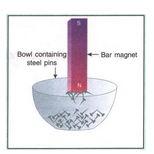To observe the attraction and repulsion between two magnets. Material required: A pair of bar magnet, three round shaped pencils. Method: Take a pair of bar magnets. Place the longer side of one of the magnets over three round shaped pencils. Now bring the north pole of one magnet near the north pole of the magnet placed on the pencils. Make sure that the two magnets do not touch each other.We see that the magnet on the pencil goes away i.e., it experience a force of repulsion (like poles repel each other). Similarly, now bring the north pole of the magnet near the south pole of the other magnet placed on pencils. We see that the magnet on the pencils get attracted towards the other magnet. It experience a force of attraction (unlike poles attract each other). This activity shows that a magnet can exert a force on another magnet without being in contact with it.  The force exerted by a magnet is an example of a non-contact force.

 To prove that a magnetic force can easily pass through wood or glass. Material required: a toy car made of iron or steel, a horse shoe, magnet, a table with glass top, a table with wooden top. Method: Place the toy car on the wooden table top. When we move the magnet under the wooden top. We see that the toy car follows the movements of the magnet.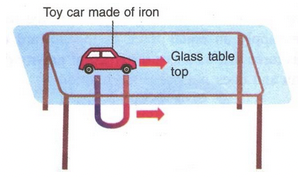Repeat the above activity with the glass table top. We again notice that here too the car follows the movements of magnet. This activity shows that a magnetic force can easily pass through substance like wood or glass. The force exerted by a magnet is an example of a non-contact force

### From above activities following conclusions can be drawn:

• A magnet exerts magnetic force on the objects made of iron or other magnets substance.
• The magnetic force can easily pass through substances like wood or glass

The force exerted by magnets on each other and metals like iron and nickel is magnetic force. When a conductor of length L carrying a current I is placed in a magnetic field of strength B.

it experiences a force given by,

F = B × I × L

If charge q is moving in a magnetic field B with velocity v, then maximum force on q is :

F = qvB

It is force exerted by a magnet or a straight current carrying conductor.

### IMPORTANT NOTE :

So far, we know of only four fundamental forces in the universe. They are gravitational force, electromagnetic force, weak force and strong interactions. We are familiar with the first two. Weak forces come into play during radioactivity. Strong interactions are responsible for holding the nucleus of an atom together. All other forces are manifestations of these fundamental forces. For example, forces such as friction between bodies, muscular action, etc. viewed on an atomic scale arise chiefly from electrical attraction and repulsion of the electrons and nuclei making up the atoms of the material.

### DIFFERENCE BETWEEN MASS AND WEIGHT :

 Mass Weight (i) It is the quantity of matter possessed by a body. It is  represented by m. (i) It is the force with which a body is attracted towards the centre of the earth. It is represented by W = mg. (ii) A mass is a constant quantity and is same (for a body) everywhere. (ii) It varies from place to place due to variation in value of g. (iii) Mass is never zero. (iii) A body has zero weight at the centre(g = 0) of the earth . (iv) Its unit is kg. (iv) Its unit is Newton. (v) It is a scalar quantity. (v) It is a vector quantity.

A force can be large or small. The magnitude tells us how large or how small is a force.

The amount or the strength of force is called its magnitude. The magnitude of force is generally shown by a straight line. It means the greater the length of line, the more is its magnitude. The direction of force is shown by placing an arrow head over the line pointing the direction in which force is acting, as shown in figure (a), (b) and (c).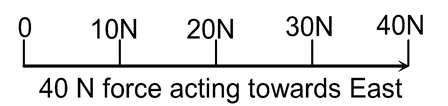(a)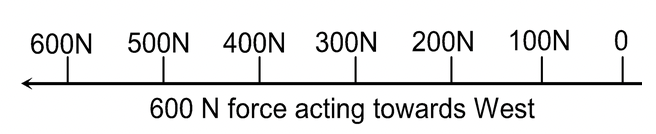(b)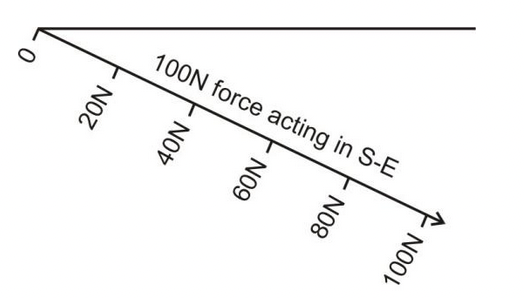(c)

Suppose a boy apply a force of 200 N to move a heavy cart but cart does not move. Now let another boy apply a force of 200 N in the same direction as shown in the figure then the cart starts moving.

The reason is that the two forces applied in the same direction, get added and produce a large resultant force (net force). This force will be given by.

200 N + 200 N = 400 N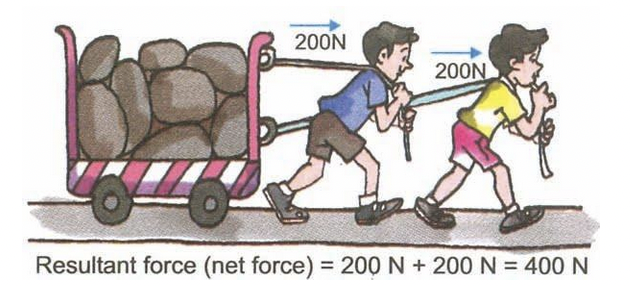### CANCELLATION AND SUBTRACTION OF FORCES:

If two boys pull the above cart in opposite directions with a force of 200 N each, as shown in figure, it is observed that cart will not move at all.

It is because the resultant force (net force) becomes zero as the forces, being equal and opposite, cancel each other.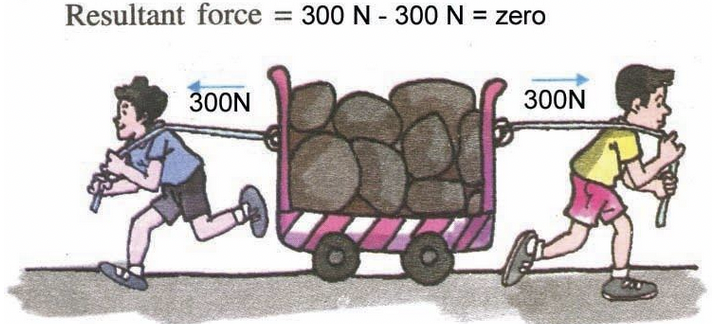If two boys pull the above cart in opposite directions but with different forces i.e., one boy apply a force of 400 N and the other boy apply a force of 300 N as shown in figure. The net force or the resultant force will be the difference of the forces and will act in the direction of the bigger force.

Thus, resultant force = (400 N − 300 N) = 100 N and will act in the direction in which A is pulling.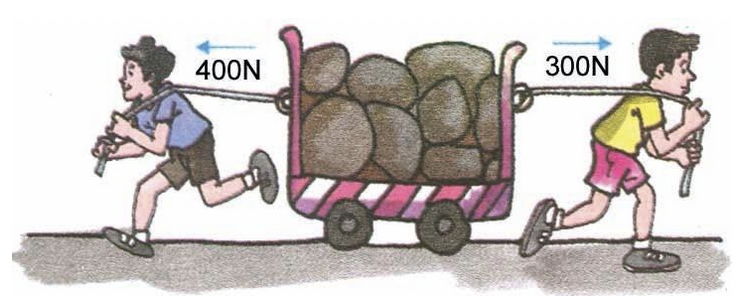Resultant force = 400N − 300N = 100

From the above examples the following conclusions can be drawn.

• When two forces are applied on the same body and at the same point but in the same direction, the resultant force (total force or net force) is equal to the sum of the individual forces acting separately. The direction of the resultant force will be the same as each of the force.
• When two forces are applied at the same point but in the opposite directions, the resultant force (total force or net force) is equal to the difference of the individual forces acting separately. The direction of resultant force will be the direction in which the larger force acts.NCERT solutions for class 8 Science prepared by Physics Wallah will help you to solve your NCERT text book exercise.